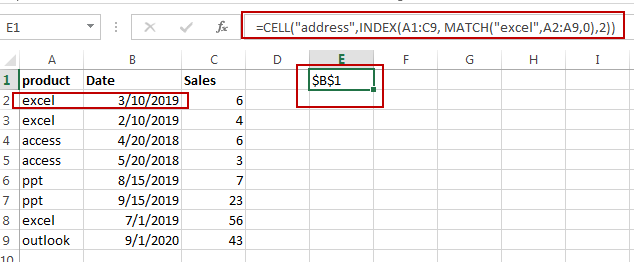This post will guide you how to lookup value and return cell reference containing that value in Excel. How do I return cell address from a lookup result with a formula in Excel.

## Return Cell Address From Lookup Result

Normally, you can use INDEX/MATCH function to lookup a value in a given range of cells, and returns cell value. If you want to return cell address instead of cell value in your formula, how to do it. In this case, you can use another function call CELL in combination with INDEX/MATCH to create a new formula to lookup a value and return cell address.

Assuming that you have a list of data in range A1:C9, and you need to look up a text string called “excel“, and return cell address of the corresponding cell value in second column. You can use the following formula:

`=CELL("address",INDEX(A1:C9, MATCH("excel",A2:A9,0),2))`

Type this formula into a blank cell and press Enter key on your keyboard.### Related Functions

• Excel CELL function
The Excel CELL function returns information about the formatting, location, size, or contents of a cell.The syntax of the CELL function is as below:= CELL (info_type,[reference])…
• Excel INDEX function
The Excel INDEX function returns a value from a table based on the index (row number and column number)The INDEX function is a build-in function in Microsoft Excel and it is categorized as a Lookup and Reference Function.The syntax of the INDEX function is as below:= INDEX (array, row_num,[column_num])…
• Excel MATCH function
The Excel MATCH function search a value in an array and returns the position of that item.The syntax of the MATCH function is as below:= MATCH  (lookup_value, lookup_array, [match_type])….

Sidebar